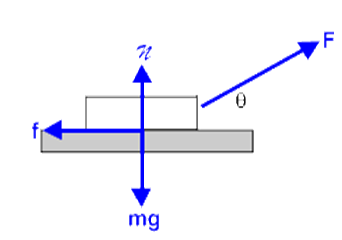Checkout JEE MAINS 2022 Question Paper Analysis : Checkout JEE MAINS 2022 Question Paper Analysis :

# Work: Factors On Which It Depends

We use terms such as overworked and hard workers to describe the effort put by a person. But what is the meaning of work and how do we quantify it? In this article, we will learn the definition of work in terms of physics and the factors on which work depends.

## Defining Work

The scientific definition of work is different in many ways from its everyday meaning. The definition of work in physics reveals its relationship to energy – whenever work is done, energy is transferred.

For a work to be done, in a scientific sense, a force must be exerted and there must be displacement in the direction of the force. With this said, we can say that

Work is the product of the component of the force in the direction of the displacement and the magnitude of this displacement.

Mathematically, the above statement is expressed as follows:

#### W = (F cos θ) d = F. d

Where,

• W is the work done by the force.
• F is the force, d is the displacement caused by the force
• θ is the angle between the force vector and the displacement vector

The dimension of work is the same as that of energy and is given as, [ML2T–2].

### Unit of Work

The SI unit of work is the joule (J), which is defined as the work done by a force of 1 Newton in moving an object through a distance of 1 meter in the direction of the force.A weightlifter lifts a barbell weighing 25 kg and displaces it from the ground by 2 m. Here, the work done upon the barbell is against gravity.

The work done upon the weight against gravity can be calculated as follows:

Work Done = (Mass × acceleration due to gravity) × Displacement

= (25 × 9.8) × 2 J

## Factors Affecting Work

Let us now consider the factors on which work done on an object by a force depends.

### Force:

Force is defined as a push or a pull that can cause any object with a mass to change its velocity and acceleration. Force is a vector quantity and has both a magnitude and a direction. If the force acting on an object is zero irrespective of the state of the object (dynamic or static) that work done by the force is zero.

### Displacement:

Displacement is a vector quantity that gives the shortest distance between the initial position and the final position of any object. If the resulting displacement in the direction of force, due to force acting on any object is zero, the net work done by that force on that object is zero. For e.g., if we push a rigid wall with all our might and still fail to displace it, then we can say no work has been performed by us on the wall.

## The Angle between the Force Vector and the Displacement VectorThe work done by a force on an object can be positive, negative, or zero, depending upon the direction of displacement of the object with respect to the force. For an object moving in the opposite direction to the direction of force, such as friction acting on an object moving in the forward direction, the work is done due to the force of friction is negative.Similarly, an object experiences a zero force when the angle of displacement is perpendicular to the direction of the force. Consider an example of a coolie lifting a mass on his head moving at an angle of 90˚ with respect to the force of gravity. Here, the work done by gravity on the object is zero.

Stay tuned to BYJU’S and Keep Falling in Love with Learning!

## Frequently Asked Questions – FAQs

### What is the standard unit of measurement for work?

The standard unit of measurement for work is Joule.

### When a body falls freely under gravity, the work done by gravity is positive or negative?

If a force acting on a body has a component in the direction of displacement, then the work done by the force is positive. Hence, when a body falls freely under the influence of gravity the work done by the gravity is positive.

### When a body slides against a rough surface, the work done by friction is positive or negative?

If a force acting on a body has a component in the direction opposite to displacement, then the work done by the force is negative. Hence, the work done by the frictional force on the body is negative.

### Superman stops a truck from moving downhill by applying force in it. Is this an example of work being done?

No. As there is no displacement no work is done. He is merely holding the car to prevent its descent down the hill.

### A string is tied to an eraser, a person holds the string and applies a tension force to the string as it made to move in a circle at a constant speed. Is this scenario an example of work being done?

For uniform circular motion, the force acts perpendicular to the direction of the motion and so the force never does any work upon the object.

1. nice

2. nitish lingampalle

thankyou such a nice explanation

3. Lakshay Fauzdaar

Helped a lot. Thanks!!

4. Vijay Raja G

Who came up with this idea of work and energy?

1. Shamoon Azam

James Prescott Joule and Hermann Ludwig Ferdinand von Helmholtz discovered and formulated the basics of what we refer to today as the law of conservation of energy: Energy cannot be created or destroyed; it can only be transformed from one form to another.